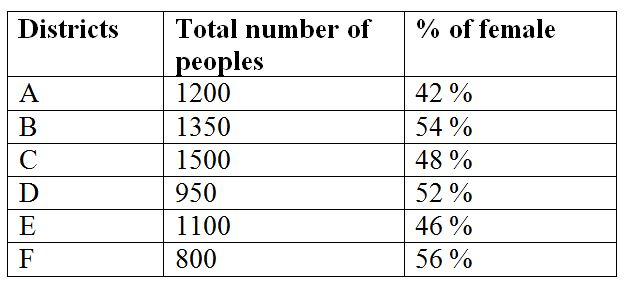# SBI Clerk/EPFO Assistant Prelims Quantitative Aptitude Questions 2019 (Day-12)

Quantitative aptitude is one of the most focusing subjects among banking aspirants.In SBI Clerk/EPFO Assistant Prelims there are 35 Marks allotted for this section. The Marks itself shows how weightage has given for Quantitative aptitude section. The SBI Clerk /EPFO Assistant 2019 exam is approaching very shortly. The exam tentatively scheduled in June 2019. Understand the importance of time and use it in the right way. As we know that SBI aptitude test questions are easy but difficult to solve within the given time limit. So practice the only solution to perform well at the time of examination. Keeping all the things in mind we are giving attention for SBI Clerk Prelims Quantitative Aptitude section. Here you can get SBI Clerk/EPFO Assistant Prelims Quantitative Aptitude Questions and answer on a daily basis. SBI Clerk Prelims Quantitative Aptitude Quiz will enhance your sped and accuracy in a self-directed way.

[WpProQuiz 6506]

Directions (Q. 1 – 5): What value should come in place of (?) in the following questions?

1) (8/9) of (27/32) of (8/15) of 15 % of 1200 = ?

a) 72

b) 84

c) 96

d) 58

e) None of these

2) (22 + 47 + 36 + 19) ÷ (7 + 5 – ? + 12) = 31

a) 24

b) 16

c) 12

d) 20

e) None of these

3) 5? = (125)2 × (625)3/4 ÷ 57 × (3125)2

a) 18

b) 12

c) 24

d) 36

e) None of these

4) 1728 × 50625 × √3136 ÷ 9= ?

a) 1340

b) 1060

c) 1120

d) 1280

e) None of these

5) 112 × 28 ÷ 16 + ? % of 400 = 531 + 132

a) 126

b) 142

c) 168

d) 150

e) None of these

Directions (Q. 6 – 10): Study the following information carefully and answer the given questions?

The following table shows the total number of peoples went for the museum from different districts in a certain week and the percentage of female among them.6) Find the ratio between the total number of female went for the museum from district A and C together to that of total number of people went for the museum from district B?

a) 17 : 23

b) 123 : 142

c) 257 : 316

d) 68 : 75

e) None of these

7) Find the difference between the total number of female went for the museum from district A and D together to that of total number of male went for the museum from district B and F together?

a) 25

b) 40

c) 70

d) 55

e) None of these

8) Find the average number of male went for the museum from district B, C and E together?

a) 680

b) 705

c) 665

d) 720

e) None of these

9) Total number of peoples went for the museum from districts C and E together is what percentage of total number of peoples went for the museum from districts A and F together?

a) 105 %

b) 130 %

c) 145 %

d) 90 %

e) None of these

10) Total number of male went for the museum from district B is approximately what percentage more/less than the total number of female went for the museum from district E?

a) 23 % less

b) 35 % less

c) 52 % more

d) 23 % more

e) 35 % more

Directions (1-5):

(8/9) * (27/32) * (8/15) * (15/100) * 1200 = x

x = 72

(22 + 47 + 36 + 19) ÷ (7 + 5 – x + 12) = 31

[124 / (24 – x)] = 31

124 / 31 = 24 – x

4 = 24 – x

x = 24 – 4 = 20

5x = (125)2 × (625)3/4 ÷ 57 × (3125)2

5x = (53)2 × (54)3/4 ÷ 57 × (55)2

5x = 56 × 53 ÷ 57 × 510

5x = 56 + 3 – 7 + 10

5x = 512

x = 12

∛1728 × ∜50625 × √3136 ÷ 9 = x

x = (12 * 15 * 56) / 9

x = 1120

(112 * 28) / 16 + (x / 100) * 400 = 531 + 169

196 + 4x = 531 + 169

4x = 504

x = 504 / 4 = 126

Directions (6-10):

The total number of female went for the museum from district A and C together

= > 1200*(42/100) + 1500*(48/100)

= > 504 + 720 = 1224

The total number of people went for the museum from district B

= >1350

Required ratio = 1224 : 1350 = 68 : 75

The total number of female went for the museum from district A and D together

= >1200*(42/100) + 950*(52/100)

= >504 + 494 = 998

The total number of male went for the museum from district B and F together

= >1350*(46/100) + 800*(44/100)

= >621 + 352 = 973

Required difference = 998 – 973 = 25

The average number of male went for the museum from district B, C and E together

= > [1350*(46/100) + 1500*(52/100) + 1100*(54/100)]/3

= > [621 + 780 + 594]/3

= > 1995/3 = 665

Total number of peoples went for the museum from districts C and E together

= >1500 + 1100 = 2600

Total number of peoples went for the museum from districts A and F together

= >1200 + 800 = 2000

Required % = (2600/2000)*100 = 130 %

Total number of male went for the museum from district B

= >1350*(46/100) = 621

Total number of female went for the museum from district E

= >1100*(46/100) = 506

Required % = [(621 – 506)/506]*100 = 22.72 % = 23 % more

3 1 vote
Rating